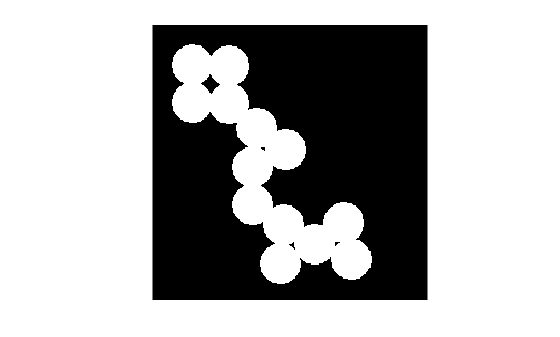# bweuler

Euler number of binary image

## Syntax

``eul = bweuler(BW,conn)``

## Description

example

````eul = bweuler(BW,conn)` returns the Euler number for the binary image `BW`. The Euler number (also known as the Euler characteristic) is the total number of objects in the image minus the total number of holes in those objects. `conn` specifies the connectivity. Objects are connected sets of `on` pixels, that is, pixels having a value of 1.```

## Examples

collapse all

Read binary image into workspace, and display it.

```BW = imread('circles.png'); imshow(BW)```Calculate the Euler number. In this example, all the circles touch so they create one object. The object contains four "holes", which are the black areas created by the touching circles. Thus the Euler number is 1 minus 4, or -3.

`bweuler(BW)`
```ans = -3 ```

## Input Arguments

collapse all

Binary image, specified as a 2-D numeric matrix or 2-D logical matrix. For numeric input, any nonzero pixels are considered to be `on`.

Data Types: `single` | `double` | `int8` | `int16` | `int32` | `int64` | `uint8` | `uint16` | `uint32` | `uint64` | `logical`

Connectivity, specified as the values `4` for 4-connected objects or `8` for 8-connected objects.

Data Types: `double`

## Output Arguments

collapse all

Euler number, returned as a numeric scalar.

Data Types: `double`

## Algorithms

`bweuler` computes the Euler number by considering patterns of convexity and concavity in local 2-by-2 neighborhoods. See  for a discussion of the algorithm used.

 Horn, Berthold P. K., Robot Vision, New York, McGraw-Hill, 1986, pp. 73-77.

 Pratt, William K., Digital Image Processing, New York, John Wiley & Sons, Inc., 1991, p. 633.